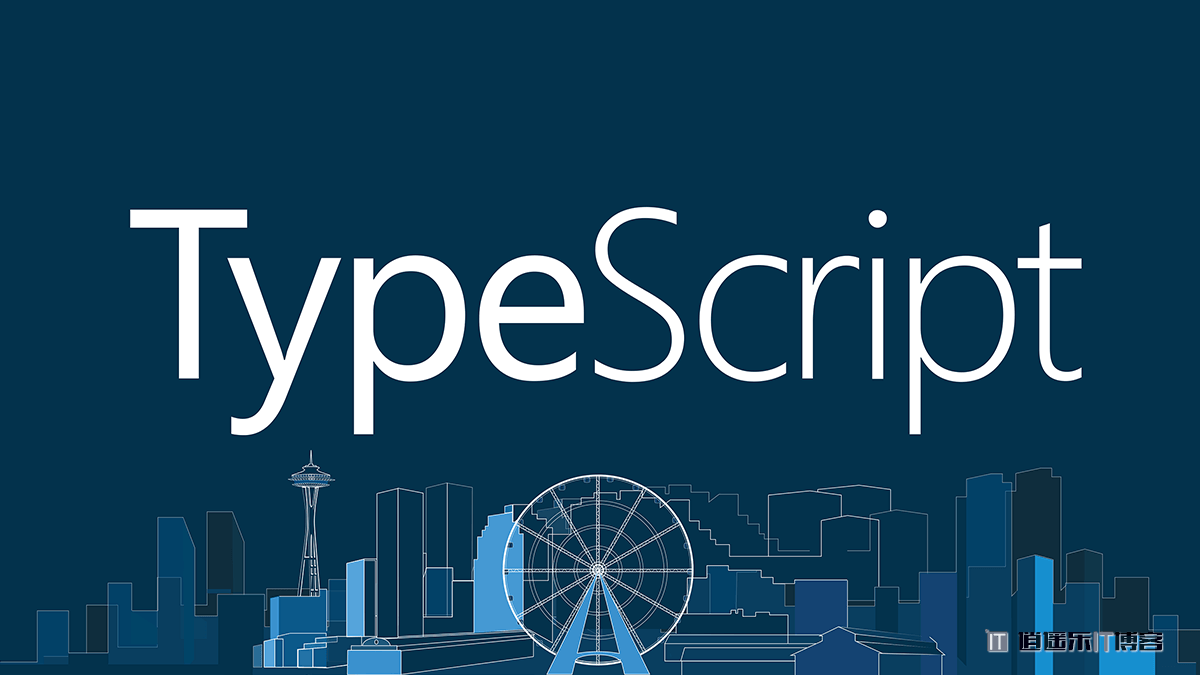# TypeScript 从零开始基础入门教程## 一、初识 TypeScript

TypeScript 是 JavaScript 的一个超集，主要提供了类型系统对 ES6 的支持，它由 Microsoft 开发，代码开源于 GitHub 上。

TypeScript 是一种由微软开发的自由和开源的编程语言。它是 JavaScript 的一个超集，而且本质上向这个语言添加了可选的静态类型和基于类的面向对象编程。

TypeScript 提供最新的和不断发展的 JavaScript 特性，包括那些来自 2015 年的 ECMAScript 和未来的提案中的特性，比如异步功能和 Decorators，以帮助建立健壮的组件。

## 二、环境搭建

``````npm install typescript -g
tsc -h   // 查看所有命令
tsc -v   // 查看版本号
``````

``````tsc hello.ts
// 把指令的 .ts 文件编译成 .js 文件。
tsc ./src/app.ts --outFile ./dist/app.js
``````

``````tsc --rootDir ./src/* --outDir ./dist --watch
``````

``````tsc --init
``````

``````tsc --watch
``````

## 三、五分钟零基础入门 TypeScript

###### 1、类型注解

TypeScript里的类型注解是一种轻量级的为函数或变量添加约束的方式。

``````let name:string = 'Jane'
``````
``````// string 是 person 函数的参数类型注解
function greet(person:string) {
return 'hello, '+person
}
greet('Jane')
greet(name)

// Argument of type 'number' is not assignable to parameter of type 'string'.
greet(20)

// Expected 1 arguments, but got 0.
greet()
``````

###### 2、接口

``````// 定义一个接口
interface Person {
firstName: string,
lastName: string
}

function greet(p:Person) {
return `hello \${p.firstName} \${p.lastName}`
}

// 测试一下
let user = { firstName:'zhang', lastName:'san' }
greet(user)
``````
###### 3、类

TypeScript支持JavaScript的新特性，比如支持基于类的面向对象编程。让我们创建一个Student类，它带有一个构造函数和一些公共字段。 注意类和接口可以一起工作。

``````// 类
class Student {
public fullName = ''
constructor(public firstName, public lastName) {
this.fullName = `\${firstName} \${lastName}`
}
}
// 接口
interface Person {
firstName: string,
lastName: string
}

// 类型注解
function greet(p:Person) {
return `hello \${p.firstName} \${p.lastName}`
}

// 测试一下
let user = new Student('zhang', 'san')
greet(user)
``````

## 四、基本数据类型

TypeScript支持与JavaScript几乎相同的数据类型，此外还提供了实用的元组、枚举、any 和 void 等。

###### 1、布尔、数字、字符串
``````// 布尔类型
let isBol: boolean = false

// 数字类型
let age: number = 20

// 字符串类型
let myname: string = 'zhangsan'
myname = "li si"
let msg = `\${myname}'s age is \${age}`
``````
###### 2、数组、元组
``````// 数组（type[] 或 Array）
let arr1: number[] = [1,2,3]
let arr2: string[] = ['1','2','3']
let arr3: Array = [4,5,6]
let arr4: Array = [['1','2'],['3','4']]

// 元组
// 元组类型允许表示一个已知元素数量和类型的数组，各元素的类型不必相同。当访问一个越界的元组元素时，会出现错误。
let x: [string, number, boolean] = ['hello', 30, true]
console.log(x,x,x)
// x = 'world' // 报错
``````
###### 3、枚举类型
``````// 枚举
enum Cate {
office = '办公用品',
car = '汽车生活',
clothe = '男装女装',
sport = '户外运动',
book = '图书音像'
}
let office_zh1 = Cate.office
console.log('enum', Cate)
``````
###### 4、any类型、void类型
``````// any类型
let noSure: any = 23.9
noSure = 'hello any'
noSure = [1,2,3]
// let noSureArr: Array = ['hello', false, 23]
let noSureArr: any[] = ['hello', false, 23]

// void类型，与any类型相反，它表示没有任何类型。
// 声明一个void类型的变量没有什么大用，因为你只能为它赋予undefined和null
let v1: void = undefined
let v2: void = null
// 当一个函数没有返回值时，你通常会见到其返回值类型是 void
function foo(arg:any): void {
console.log('该函数的返回值类型为void')
}
``````

## 五、函数

###### 1、函数声明
``````// 一个函数有输入和输出，要在 TypeScript 中对其进行约束
function sum(x: number, y: number): number {
return x + y
}
sum(1,2)
// 输入多余的（或者少于要求的）参数，是不被允许的
// sum(1)
// sum(1,2,3)
``````
###### 2、函数表达式
``````let sub = function(x: number, y: number): number {
return x - y
}
sub(10,5)

// 还可以使用接口的方式来定义一个函数需要符合的形状
interface SearchFunc {
(source: string, subStr: string): boolean
}
let search: SearchFunc = function(source: string, subStr: string) {
return source.search(subStr) !== -1
}
``````
###### 3、可选参数
``````// 用 ? 表示可选的参数，可选参数必须接在必需参数后面，可选参数后面不允许再出现必需参数了。
function foo(a: string, b?:string): string {
if(b) return a+b
return a
}
foo('hello')
foo('hello','world')
``````
###### 4、参数默认值
``````// TypeScript 会将添加了默认值的参数识别为可选参数
// 此时，可选参数不必一定要在必需参数后面
function bar(a:string='hello', b:string) {
return a +b
}
bar(undefined,'world')
bar('hi', 'lisi')
``````
###### 5、剩余参数
``````// 函数剩余参数可以使用 ...rest 的方式获取函数中的剩余参数（rest 参数）
function push(arr:any[], ...rest:any[]): any[] {
return [...arr, ...rest]
}
push(, 1,2,3,4)
``````
###### 6、函数重载
``````function reverse(x: number): number
function reverse(x: string): string
function reverse(x: number | string): number | string {
if (typeof x === 'number') {
return Number(x.toString().split('').reverse().join(''))
} else if (typeof x === 'string') {
return x.split('').reverse().join('')
}
}
// 我们重复定义了多次函数 reverse，前几次都是函数定义，最后一次是函数实现。
// TypeScript 会优先从最前面的函数定义开始匹配，所以多个函数定义如果有包含关系，需要优先把精确的定义写在前面。
reverse(100)
reverse('hello')
``````

## 六、接口

``````interface Person {
name: string,  // 必须属性
age: number,   // 必须属性
mobile?: string,   // 可选属性
[propName: string]: any,  // 任意属性
}

// 赋值的时候，变量的形状必须和接口的形状保持一致
let tom: Person = {
name: 'tom',
age: 30,
mobile: '110',
gender: 'male',
role: 1
}
``````
• 注意1：使用 [propName: string] 定义了任意属性取 string 类型的值。一旦定义了任意属性，那么确定属性和可选属性的类型都必须是它的类型的子集。
• 注意2：只读的约束存在于第一次给对象赋值的时候，而不是第一次给只读属性赋值的时候。

## 七、泛型

``````function print(arg: T): void {
console.log('arg', arg)
}
print('hello')
print(100)
print(1.2345)
``````
``````function add(x: T, y: T, z: T): void {
console.log(x, y, z)
}
``````

1、支持使用多个泛型

``````function swap(tup:[T,U]): [U,T] {
return [tup, tup]
}
swap([100, 'hello'])   // ['hello', 100]
interface MyU { a: string; b: number }
swap([true, {a:'hi',b:11}])  // [{a:'hi',b:11}, true]
``````

2、泛型约束

``````interface LengthType {
length: number
}
function printLength(arg: T): number {
// 为什么要进行泛型约束呢？因为入参的数据类型不确定，它可能没有length属性
return arg.length
}
printLength('hello')   // 5
printLength>([1,2,'a','b'])  // 4
``````

3、泛型类

``````class Dog {
name: T
constructor(name: T) {
this.name = name
}
sayHi(): T {
return this.name
}
}
const dog = new Dog('dahuang')  // 调用constructor()
dog.sayHi()
``````

4、泛型接口（泛型类型）

``````interface Tfn1 {
(x: T, y: T, z: T): T
}
const tf1: Tfn1 = function(x: number, y: number, z: number): number {
return x * y * z
}
tf1(1,2,3)
const tf3: Tfn1 = function(x: string, y: string, z: string): string {
return x + y + z
}
tf3('a','b','c')
``````
``````interface Tfn2 {
(x: T, y: T, z: T): void
}
const tf2: Tfn2 = (x:T, y:T, z: T): void => {
console.log(x, y, z)
}
// const tf2: Tfn2 = function (x:T, y:T, z: T):void {
//  console.log(x, y, z)
// }
tf2('a','b','c')
tf2(10, 20, 30)
``````